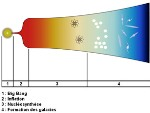# Gravity

## The problem of gravityAutomatic translation Category: galaxies
Updated June 01, 2013

In 1665 a man was sitting under a tree when suddenly he saw a legendary apple falling right in front of him. With the fall of the apple Isaac Newton revolutionized the whole image of the Universe.
In a bold hypothesis for his time, he asserts that the force that pulls the apple to the ground is the same one that keeps the Moon around the Earth.
In 1686 he managed to express his theory as a mathematical equation. The force is equal to the masses of two objects, divided by the square of the distance between them, which must integrate the universal gravitational constant which determines the overall strength of gravity.
Although Newton's theory has allowed us to go to the moon, this theory is not entirely fair.
During the Apollo program astronomers have left on the Moon mirrors that reflect all light rays pointed above. These mirrors were used to measure the Earth-Moon distance with great precision, the order of cm. Even if it's still a very complicated experience the accuracy is 1 to 3 cm on 400 000 km. These results show that the orbit of the Moon is different from that predicted by Newton. Newton's law with the calculated distance of the Moon is false, a dozen meters.
Newton gave us an equation to calculate the severity but has no idea how it works, besides, he thinks that this force is divine. In Newton's universe is empty space and galaxies and stars influence each other. In one of Einstein it consists of a four-dimensional fabric called it space-time.

In other words it is the galaxies, stars and planets that twist and distort space-time.
The greater the mass, the larger one is close to an object and the space time is distorted. He hoped the four-dimensional fabric of space-time would allow him to speak simply of objects moving along the surface of this space-time.
As the surface of a trampoline, the fabric is stretched and distorted united by heavy objects such as planets and stars and it is this distortion or curvature of space-time, which creates what it feels like gravity.
He calls this new picture of gravity, general relativity. This image of seriousness can solve the riddle of the strange cosmic objects that we transmit multiple views of a single galaxy.
They are gravitational lenses. A gravitational lens or gravitational mirage is a very massive object, a galaxy cluster instance, which is located between an observer and a distant source of light.
The gravitational lens prints a strong curvature in space-time, which has the effect of deflecting all light rays that pass near it, thereby distorting the images received by the observer.
This amplification of the brightness of a distant celestial object by a massive star in front, was predicted by the theory of general relativity in 1917.
Massive objects therefore modify the geometry of space and time, in their neighborhood.## The secrets of gravity

Einstein predicted that gravity is more significant, as time unfolds slowly over the gravity low over time flies. We also know that the time and space are intimately linked. The farther from Earth, the greater the severity is low, the gravity is different in space than on Earth. Gravity warps as time, space time goes a little faster than on Earth. GPS operation described exactly this curvature of space-time. For the GPS to work properly, the clocks of the 31 GPS satellites, must be perfectly synchronized with time on Earth. At 20 200 km altitude, if the clocks of the satellites are not synchronized, the values given by the GPS derived from 10 to 12 km per day. A time correction is made to fit the GPS to the effects of gravity. But in the cosmos, where the orbiting massive objects in the universe, space and time shrink even more as a result of the huge gravitational forces. A pulsar is a dead star, a dwarf extremely dense 10 km in diameter, which turns on itself 10 to 1000 times per second. In some areas there are double pulsars that orbit one around the other 5 to 1000 revolutions per second.

According to Einstein, these violent cosmic phenomena create gravitational waves that stretch and contract the space and time, distorting our physical reality. This cosmic phenomenon described by Einstein has not been observed. But how gravity works it objects to the scale of the infinitely small?
Einstein was not able to explain, the theory does not work at very small distances, as in the world of subatomic particles.
Since then, theorists of the infinitesimally small trying to understand how gravity by attempting to recreate the Big Bang in particle accelerators.
Particle physicists seek, through the LHC, this hypothetical graviton. This quest is not easy because we touch, the invisible dimensions of our reality.

### Image: The LHC is built in a tunnel of 3 meters in diameter and 26.659 km in circumference, should revolutionize our understanding of the universe, from the infinitely small to the infinitely large.1997 © Astronoo.com − Astronomy, Astrophysics, Evolution and Earth science. Contact    Mentions légalesDoes the black mater exist?Non-baryonic dark matter...